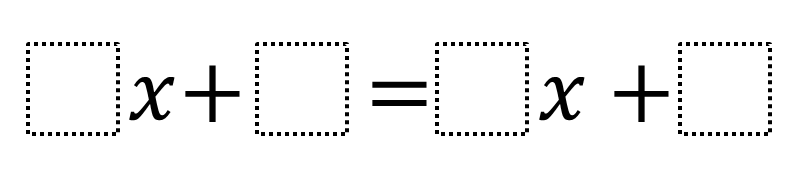# Create an Equation with a Given Solution

Directions: Using the digits 0 to 9 at most one time each, fill in the boxes to write three equations whose solution is -1/2.### Hint

What does it mean to be a solution of the equation?
How would you check if a value is a solution to an equation?
What kind of numbers would you put in for the coefficients of x?

There are many arrangements. Here is one set.
2x + 5 = 4x + 6
2x + 7 = 4x + 8
8x + 5 = 4x + 3

Source: Daniel Luevanos

## Difference of Squares and Sum of Cubes

Directions: Using the digits 0 to 9 at most one time each, place a digit …

1.2x + 5 = 4x + 6
2x + 7 = 4x + 8
8x + 5 = 4x + 3

2.x+4=3x+5

x+6=3x+7

2x+5=4x+6

3.2x+3=4x+4

4x+2=8x+4

2x+1+4x+2

4.5x+7=3x+6
3x+10=x+9
6x+14=4x+13

5.6.2x+1=4x+2
6x+3=8x+4
8x+3=4x+1

7.5x+7=3x+6
8x+3=4x+1
4x+2=8x+4

8.sophia antogiovanni

2x+4=4x+5
2x+3=4x+4
4x+5=8x+6

9.2x+3=4x+4

10.6x-4=-2+6x

11.2x+4= 4x+5

12.4x+6=6x+7
6x+7=8x+8
8x+8+10x+9

13.2x + 6 = 4x + 7
2x + 7 = 4x + 8
8x + 5 = 4x + 3

14.7x+8=5x+7
x+6=3x+7
4x+1=2x+5

15.5x+7=7x+8
4x+6=6x+7
3x+5=5x+6

16.3x+1=6x+2

•*4x+1=6x+2

17.7x+8=5x+7
x+6+3x+7
* 4x+6=6x+7

18.7x+8=5x+7
x+6+3x+7
4x+6=6x+7

19.2x + 5 = 4x + 6
2x + 7 = 4x + 8
8x + 5 + 4x + 3

20.x+9 = 3x+10
4x+9 = 2x+8
6x + 10 = 4x +9

21.6x+3=4x+2
x+8.5=2x+9
2x+7=-8x+2

22.3x+3=x+2
4x+4=2x+3
5x+5=3x+4

•3x+5=x+4
4x+6=2x+5
5x+7=3x+6

23.4x+6=8x+8
8x+9=4x+7
2x+5=4x+6

24.2x+5=4x+6
3x+1=6x+5
2x+4=4x+5

25.5x+7=3x+6
6x+7=8x+8
4x+2=8x+4

•6x+6=2x+4
3x+1=5x+2
5x+4=3x+3

26.6x+3=4x+2
3x+1=6x+5
8x+5+4x+3

27.6x+3=4x+2
3x+1=6x+5
8x+6+4x+4

28.2x+1=4x+0
6x+2=8x+1
3x+1=x+2

29.8x-1=10x
4x-1=2x-2
12x-1=16x+1

30.6x-4=4x-5
8x+9=4x+7
12x-6=10x-7

31.william hockstein

-6-x=-5+x
x=-8(2)/32
8+x=20+x-12

32.2x+3=4x+4
4x+2=8x+4
2x+1=4x+2

33.x+3 = 2x+5

•6x+ 1= 3x+ 2
1.5x+ 2= 3x+4

34.4x+6=3-2x
1041x+500=41x
5x/2+27x/2=-9x/2+10

35.6x + 2 = 10x + 4
10x + 3 = 16x + 6
2x + 3 = 4x + 4

36.8x+7=4x+5
8x+2=6x+1
6x+7=2x+5

37.4x+3=2x+2
8x+9=6x+8
3x+2=x+1

38.6x+5=8x+6
10x+10=12x+11
36x+8=38x+9

39.3x+5=5x=6
4x+5=3x+3
8x+8=7x+6

40.4x+2=8x+4
3x+5=5x=6
8x+2=6x+1

41.x + 2 = 3x +3
-11x – 6 = 3x +1
5x +8 = 3x + 7

•just realized 2nd one had an 11

changed it to – 9x – 6 = 5x +1

42.-7x-5=9x+3
6x+8=4x+7
-3x-6=7x-1

43.2x+3=6x+5
8x+4=6x+3
6x+9=4x+8

44.2x+3=4x+4
-2x-2=-4x-3
3x+12=x+11

45.2x+5=4x+6.
4x+4=2x+3.
3x+1=6x+2

46.Create an Equation with a Given Solution
Directions: Using the digits 0 to 9 at most one time each, fill in the boxes to write three equations whose solution is -1/2
_x + _ =_x + _ -> original problem
6x +9 =4x +8 -> my attempt

47.4x + 7 = 6x + 8 —> -1 = 2x —> -0.5 = x
2x + 3 = 6x + 5 —> -2 = 4x —> -0.5 = x
4x + 5 = 8x + 7 —> -2 = 4x —> -0.5 = x

48.49.